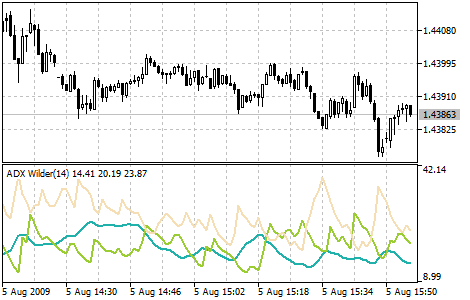MetaTrader 5 HelpPrice Charts, Technical and Fundamental AnalysisTechnical IndicatorsTrend IndicatorsAverage Directional Movement Index Wilder

# Average Directional Movement Index Wilder

Average Directional Movement Index Wilder (ADX Wilder) helps to determine if there is a price trend. This technical indicator is constructed as a strict correspondence with the algorithm described by Welles Wilder in his book "New concepts in technical trading systems".

Trading rules of this indicator are described in the section "Average Directional Movement Index".## Calculation

First positive (dm_plus) and negative (dm_minus) changes at each bar are calculated, as well as the true range tr:

If High(i) - High(i-1) > 0  dm_plus(i) = High[(i) - High(i-1), otherwise dm_plus(i) = 0.
If Low(i-1) - Low(i) > 0  dm_minus(i) = Low(i-1) - Low(i), otherwise dm_minus(i) = 0.

tr(i) = Max(ABS(High(i) - High(i-1)), ABS(High(i) - Close(i-1)), ABS(Low(i) - Close(i-1)))

Where:

High(i) — maximal price of the current bar;
Low(i) — minimal price of the current bar;
High(i-1) — maximal price of the previous bar;
Low(i-1) — minimal price of the previous bar;
Close(i-1)  — close price of the previous bar;
Max (a, b , c) — maximal value out of three numbers: a, b and c;
ABS(X)  — value of the number X absolute in its module.

After that smoothed values are calculated: Plus_D(i), Minus_D(i) and ATR():

Where:

SMMA(X, N, i) — Smoothed Moving Average by values of X series on the current bar;
Period_ADX — number of periods used for calculation.

Now Directional Movement Index - DX(i) - is calculated:

DX(i) = ABS(Plus_D(i) - Minus_D(i))/(Plus_D(i) + Minus_D(i)) * 100

After preliminary calculations we obtain the value of the ADX(i) indicator on the current bar by smoothing DX index values: